# Numb3rs 305: Traffic

The main mathematical topic in this episode is the question of determining whether something is random or not. There is also a brief discussion of choosing road widths to optimize traffic flow, which is mentioned in a Tangent.

## Randomness

One of the main mathematical issues in this show was determining whether some particular data set was actually random. Of course, this is impossible to do with absolute certainty. For example, if someone told you they had rolled a dice 100 times and gotten a 1 every time, it would be reasonable to say that either they were lying or the dice rolled wasn't random. However, it is possible to roll a 1 100 times in a row, so one can't be completely sure that the person was lying or that the dice isn't fair. What we would like is a mathematical procedure that takes some data as input and then outputs a number that indicates how likely it is that the data is random. For example, given n dice rolls one could take the sum of all the rolls. Then if n is large, say over 50, in almost all cases the sum of the dice rolls would be close to 7n/2. However, in the example above, the sum is 100, which is far from 350. This would indicate that the data is unlikely to be random. (The pedantic reader might complain that the proper singular/plural forms are die/dice, respectively. The choice of the plural form in all uses was made due to the multiple definitions of the word "die". Hopefully this will not be too distracting.)
Activity 1: This is a partner activity. Both you and your partner should follow the following instructions seperately.
1. Write down a series of 20 dice rolls, trying to make them seem random.
2. Find a dice and roll it 20 times, recording the results on a seperate piece of paper.
3. Give both pieces of paper to your partner (and take their pieces of paper). Try to guess which sequence is random and which was created by your partner.

Let's study what we just did a little more carefully. First, let's look at one roll of the dice. This is an example of a random variable. For our purposes, we will define a random variable as set of pairs (number, probability) such that the sum of all the probabilities is 1. The first number in each pair is supposed to be the outcome of some random event, and the second number is the probability of that outcome. For example, we could describe the random variable corresponding to a fair dice as X = {(1,1/6), (2,1/6), (3,1/6), (4,1/6), (5,1/6), (6,1/6)}. The random variable corresponding to the unfair dice that was rolled in the first paragraph seems likely to be X = {(1,1)}, i.e. the only possibility is to roll a 1. In the above paragraph we said that the sum of the rolls we were testing was 100, which was far from the expected value of 350. Now can we describe this in a more rigorous way? Yes, using the mathematical idea of expected value (this is one of the cases where the name for a mathematical concept means almost exactly what it does in normal English). First we define the expected value, or expectation, of one random variable X. The formula isThe equation has a couple funny symbols in it, but in words this means that you take each possible outcome, multiply it by the probability that it occurs, and then sum all of these numbers up. For a fair dice with 6 sides, this leads to E(X) = 1/6 + 2/6 + 3/6 + 4/6 + 5/6 + 6/6 = 3.5. Taking the expectetation of a random variable is essentially figuring out what its average value is.

Now that we've defined expectation, how can we apply it to multiple dice rolls, or random variables in general? We'd like to figure out the expected value of the sum of several dice rolls. To do this, we'll define addition of random variables in general, and then see how addition interacts with expectation.

In the episode Larry mentions the quote "Mathematicians are machines for turning coffee into theorems," which he attributes to Renyi, but has also been attributed to Erdos, a famously eccentric Hungarian mathematician. He said it because mathematicians take coffee as input and then are supposed to output theorems.
Activity 2:
1. If expectation is supposed to correspond to averages, what do you think the expectated sum of rolling two fair dice is?
2. What do you think the most common value of the sum of two fair dice is?
3. Do Activity 2 in Running Man. How do your answers there compare with the answers to the two previous questions?
4. Do you have a guess as to how expectation and summation of random variables will interact?
Now let's define the sum of two random variables X,Y. First we'll give the formula and then explain what it means in words.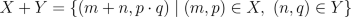This formula probably contains some symbols you aren't familiar with, so we'll explain them. First, the outer squiggly braces mean that we are creating set. In general, the expression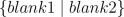refers to a set. The elements of the set are described in blank 1, and these elements are subject to the restrictions listed in blank 2. In this case, the elements are pairs (m+n,pq), and the restrictions are that (m,p) must be an possible event for the random variable X and (n,q) must be a possible event for the random variable Y. An observant reader will notice that we are making an assumption here that hasn't been stated yet. We are implicitely assuming that the probability of the event "m and n both happen" is the product pq. However, this is only the case if the random variables X, Y are completely unrelated to each other, or independent. For example, if X is 1 roll of a dice, and Y is a second roll of a dice, then X and Y are independent, but if X is the sum of a red and a blue dice and Y is the value of the red one, then X and Y aren't independent. From now on we'll assume that all of our random variables are independent. Let's look at an example where X, Y are both random variables corresponding to a flip of a fair coin with sides labelled by 1 and 2. Since the possible outcomes of the new random variable X + Y correspond to pairs of outcomes, one each from X and Y, we can write down the possible outcomes for X + Y in a table.
 (1,1/2) in X (2,1/2) in X (1,1/2) in Y (2,1/4) (3,1/4) (2,1/2) in Y (3,1/4) (4,1/4)
Notice that the outcome 3 occurs twice, each time with probability 1/4. This is the same as if 3 occured once with probability 1/2. Therefore, we can represent the new random variable X + Y as {(2,1/4),(3,1/2),(4,1/4)}. Now if we calculate expectations, we get E(X) = 1*(1/2) + 2*(1/2) = 1.5 and E(X + Y) = 2*(1/4) + 3*(1/2) + 4*(1/4) = 3. In this case, E(X) + E(Y) = E(X + Y). Is this always true? It's not too hard to show that it is, although as before the notation might be a little confusing.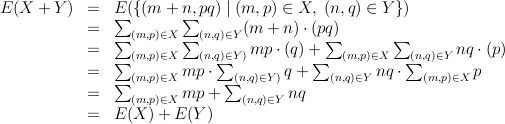Going from the first to the second line (and the fifth to sixth) we use the definition of expectation, and going from the fourth to the fifth we use the fact that the sum of all the probabilities for any random variable is 1. The rest is properties of arithmatic, i.e. multiplication and addition distribute over each other. Now we have completed the goal stated in the first paragraph. If we roll a dice 100 times then the expected value of the sum of the rolls is 100E(X) = 350. This would indicate that if the sum of the rolls is 100, then the dice probably isn't fair.

However, this isn't the whole story. For example, if you said you got 50 1's and 50 6's, then the sum would be 350, which is exactly what the expected value is. We need some other kind of measurement to go along with the expected value measurement. One candidate is the variance. This is supposed to measure how different the values are from the average value, and is defined as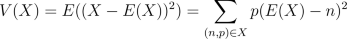. Notice that in the first equation we are subtracting E(X) from X. This is the same as adding the random variable {(-E(X),1)} to X. (E(X) is a constant, because it doesn't depend on the possible outcomes of X, which we are summing over.) Now when we square the random variable (X - E(X)) we aren't actually multiplying two random variables together, we're just squaring the numbers that are the possible outcomes. More precisely, the square is defined as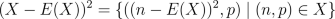.

The obvious next question is how does variance interact with addition? If the random variables are independent (which means that the outcome of one doesn't depend at all on the outcome of the other), then variance is also additive, as we show below. Throughout the mu's with the subscript refer to the expectation of X and Y.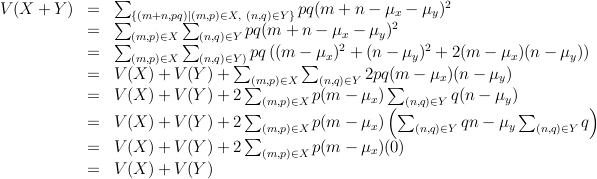Plugging into the formula, if X is the random variable corresponding to the roll of 1 dice, then V(X) = 1/6(2.5^2+1.5^2+.5^2+.5^2+1.5^2+2.5^2) = 3 - 1/12. (Unfortunately it isn't an integer or very nice fraction.) This gives us another way to test if a sequence of dice rolls is random. If someone claimed they rolled 50 1's and 50 6's, then the sum of their rolls would be 350, which is exactly the expected value of the sum of 100 dice rolls. However, the variance would be 50*(6-3.5)^2 + 50*(1-3.5)^2 = 625, but the variance of the random variable corresponding to the sum of 100 dice rolls would be 300 - 25/3, or about 292, which is much smaller. Now we have two ways to tell if a sequence isn't random. Of course, these will miss some ways that sequences can fail to be random, for example, the sequence of rolls 1,2,3,4,5,6,1,2,3,4,5,6,... will appear to be random using these tests. Of course, this sequence of rolls will be impossible to reject as nonrandom by any test which doesn't depend on the order of the rolls, since the distribution of the numbers is exactly what it should be.

We've talked about addition of random variables, but we haven't talked about multiplying them by a scalar quantity (this would be like multiplying the values on a dice by some fixed number). If c is a fixed number and we write cX to denote this process, then we can figure out what happens when we take the expectation and variance of cX.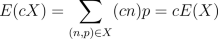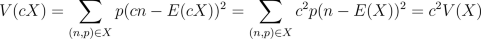Therefore, if instead of adding up 100 dice rolls we wanted to average 100 dice rolls, our expectation would remain the same but the variance would decrease. Specifically, if X_i corresponds to the roll of dice number i, then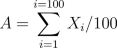is the average of 100 rolls, and E(A) = 3.5 and V(A) = (3-1/12)/100.

In the episode Charlie briefly mentions that dynamic fluid flow equations (which are PDEs, partial differential equations) can help optimize the widths of roads, speed limits, etc. in systems of roads. We won't discuss this here because it involves advanced calculus, but there is an interesting and un-intuitive fact that we will mention. If you have a system of roads and then add a road to it, it can actually increase average travel times of the drivers on the road! A short explanation of why this can happen is as follows: each driver is trying to minimize his/her total driving time and doesn't care about the driving times of other drivers. This means he/she doesn't care about the average driving time very much. Since the only optimization occuring is the optimization performed by the individual drivers and they don't care about average driving time, there's no reason to expect that average driving time will be minimized. This is very similar to the Prisoner's Dillemma, where the prisoner's total utility is highest if they cooperate, but if they operate selfishly they do not achieve this maximum total utility.
One reason the concept of adding random variables is important is a deep mathematical theorem called the Central Limit Theorem. The basic idea of this theorem is that the sum of many copies of the same random variable is approximated very well by the Gaussian Distribution, or to use plainer English, looks like a Bell curve. This is nice because the Gaussian distribution has the very nice property that it is completely determined by its expected value and variance. Furthermore, if we call the square root of the variance a standard deviation, then if we evaluate (also called sampling) the Gaussian distribution, 68%, 95% and 99.7% of the time the value will be within 1, 2, and 3 standard deviations of the expected value, respectively. (If X is the random variable corresponding to rolling a dice, then sampling X means rolling the dice and seeing what the value is.) This gives us a more quantitative way of declaring that some sequences of rolls are unlikely to be random. For example, if we roll a dice 20 times, then then expected average of these rolls will be 3.5 and the variance of the average will be (3-1/12)/20, which is about .13. Then averages of above 3.63 or below 3.37 will only occur 32% of the time, and averages of above 3.89 or below 3.11 will only occur .3% of the time.
Activity 3:
1. Find the expected value and variance of the random variables corresponding to the sum and average of 20 dice rolls.
2. Take the lists from activity 1 and calculate each of the values in the previous problem for both lists. Which list, the random or human-generated one, has "better" values (closer to what you expect)?
3. Would these tests have helped you to predict which list was random?